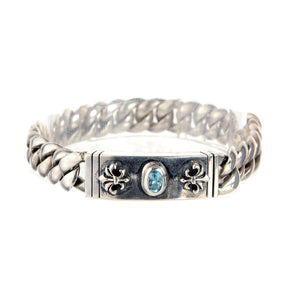Liquid error (image-logic line 10): divided by 0Liquid error (image-logic line 10): divided by 0
Liquid error (image-logic line 10): divided by 0
Liquid error (image-logic line 10): divided by 0
Liquid error (image-logic line 10): divided by 0
Liquid error (image-logic line 10): divided by 0
Liquid error (image-logic line 10): divided by 0

# Silver Bracelet Oval STONE and LILIES Chain

Elfcraft

Product SKU: 200.004.1

• €325,00
Unit price per
Tax included.

Plain curb chain xs and stone with 2 Lilies boxlock